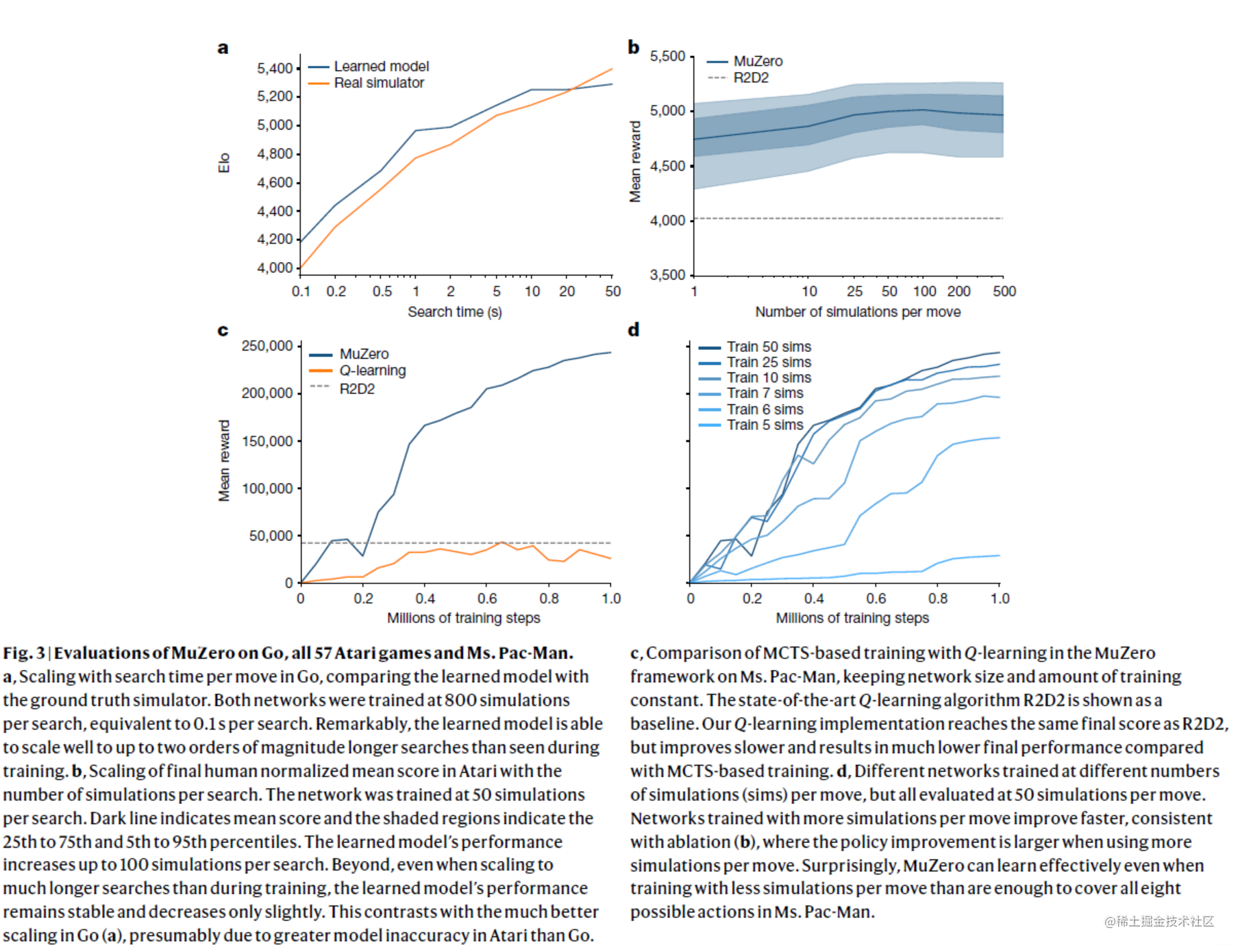# 【Nature论文浅析】基于模型的AlphaGo Zero

• 论文题目：Mastering Atari, Go, chess and shogi by planning with a learned model

## 所解决的问题？

规划planning一直都是人工智能研究领域中，人们追逐的一个比较难的研究点，基于树的规划算法，像AlphaGo这类算法已经取得了巨大的成功，然而基于树模型的规划算法需要一个完美的环境模型，这个条件在真实的世界中是很难被满足的。

## 背景

基于模型的强化学习方法先学一个环境模型，然后基于所学的这个环境模型去规划能够解决无法在真实环境交互过多的问题。以往的经典的规划算法往往都会依赖被控对象的模型，这样对于实际地落地就存在很大的阻碍。现在做的比较好的基于模型的强化学习算法，都不会聚焦在重建整个的环境，也就是说一般不会重构整个的观测序列。像abstract mdplatent space这类方法都能够比较高效地做值函数的估计。

## 所采用的方法？

MuZero是基于AlphaZero的改进版本。MuZero扩展AlphaZero到单个智能体领域以及非终止奖励的控制对象上。

算法主要的思想是预测未来，主要是预测那些可以直接用来规划未来的数据，比如预测未来的值函数。之后就可以基于预测数据进行规划。

• MuZero算法

具体做法是：模型接收一个观测数据(比如游戏的一帧像素)，然后将其编码到隐藏状态空间。在隐藏状态空间可以通过给定动作和自回归的方式进行学习和训练。在每一步，模型需要产生(或者说去预测)一个策略，一个值函数(预测的累计奖励)，和一个即时奖励的预测(当前步的奖励预测)。模型端到端直接训练好，并且作者这里并不采用dreamerplanet学习环境模型的方法，认为模型没有必要具有从隐状态复原到原像素这种能力。隐状态只要能够去正确地估计策略、值函数和即时奖励即可

![](img-blog.csdnimg.cn/20210117160… =700x)

给定一个时间步$t$，对于每一步$k=0,\cdots,K$步，一个带有$\theta$参数的模型$\mu_{\theta}$，基于给定的过去的观测数据条件$o_{1}, \cdots,o_{t}$和未来的动作$a_{t+1}, \cdots, a_{t+k}$(其中$K>0$)来预测未来的：

策略

$p_{t}^{k} \approx \pi\left(a_{t+k+1} \mid o_{1}, \ldots, o_{t}, a_{t+1}, \ldots, a_{t+k}\right)$

值函数

$v_{t}^{k} \approx \mathbb{E}\left[u_{t+k+1}+\gamma u_{t+k+2}+\ldots \mid o_{1}, \ldots, o_{t}, a_{t+1}, \ldots, a_{t+k}\right]$

即时奖励

$r_{t}^{k} \approx u_{t+k}$

其中$u$是真实地观测奖励，$\pi$是策略，$\gamma$是折扣因子。

说白了就是拿到过去的观测数据，编码到当前的隐藏状态，然后再给定未来的动作，就可以在隐藏状态空间中进行规划了

1. 想要实现上述功能，与基于模型的算法一样，两部走；学环境模型和策略规划。

环境模型需要提供的是：1. 状态转移；2. 每个节点允许搜索的动作(减少搜索空间)；3. 终止节点。用神经网络来做就好了，环境模型其实是由两部分组成，表示模型和动态模型$g_{\theta}$

$r^{\mathrm{k}}, s^{k}=g_{\theta}\left(s^{k-1}, a^{k}\right)$

表示函数$h_{\theta}$对过去的观测进行编码$s^{0}=h_{\theta}\left(o_{1}, \ldots, o_{t}\right)$，得到当前根节点$s^{0}$。给定这样一个模型之后，对于一个未来假设的轨迹$a^{1}, \ldots, a^{k}$，和给定的过去的观测$o_{1}, \ldots, o_{t}$

1. 策略用的是与AlphaGo Zero一样的MCTS策略，需要搜索一个策略$\pi_{t}=\mathrm{P}\left[a_{t+1} \mid o_{1}, \ldots, o_{t}\right]$和一个值函数$v_{t} = \mathbb{E} \left[u_{t+1}+\gamma u_{t+2}+\ldots \mid o_{1}, \ldots, o_{t}\right]$loss由策略、值和奖励这三部分的loss组成：

$l_{t}(\theta)=\sum_{k=0}^{K} l^{\mathrm{p}}\left(\pi_{t+k}, p_{t}^{k}\right)+\sum_{k=0}^{K} l^{\mathrm{v}}\left(z_{t+k}, v_{t}^{k}\right)+\sum_{k=1}^{K} l^{\mathrm{r}}\left(u_{t+k}, r_{t}^{k}\right)+c\|\theta\|^{2}$

## 取得的效果？

• 实验结果总之就是很厉害啦！## 所出版信息？作者信息？Julian Schrittwieser谷歌大脑软件工程师！AlphaGoAlphaZero 的项目组成员。